## 27 Dec 2018

### Wildberger (32) WildTrig: Intro to Rational Trigonometry, 32: “Projective Geometry and Perspective”, summary

[Search Blog Here. Index tabs are found at the bottom of the left column.]

[Central Entry Directory]

[Mathematics, Calculus, Geometry, Entry Directory]

[Norman Wildberger, entry directory]

[Wildberger, Wildtrig, entry directory]

[The following is summary of Wildberger’s video lecture. You will find that he is a supremely talented teacher. Any mistakes are my own, as I am not a mathematician. Geometry graphics not from the video are made using Geogebra. Bracketed comments are my own and are not to be trusted.]

Norman J. Wildberger

Course Series

WildTrig: Intro to Rational Trigonometry

32

“Projective Geometry and Perspective”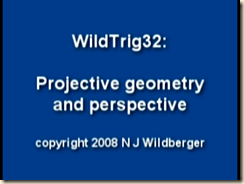(Image source: Wildberger)

Brief summary:

(32.1) In this lesson we will examine how projective geometry arose from artists’ work on perspective. (32.2) Renaissance painters discovered techniques that implement perspective so to accurately depict the spatial proportions of a three dimensional object upon a two-dimensional surface, as seen from a fixed point of view. The canvas is a two-dimensional “picture plane” set between the artist’s eye and the three-dimensional object placed at a distance. There are lines going from the artist’s eye through the picture plane and to the various parts of the external object. By delineating the depiction according to the places of canvas-intersection of the lines of sight going to the object’s various parts, the object is depicted on the canvas with its proper visual proportions for that perspective.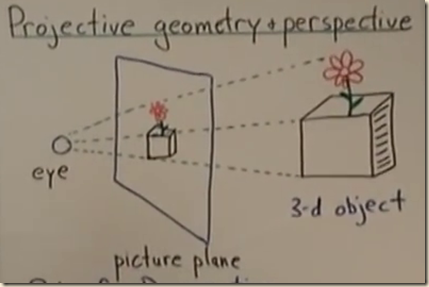(Image source: Wildberger)]

(32.3) The Renaissance painters discovered geometrical rules governing perspective painting. Rule 1: The image of a straight line is always a straight line. (32.4) Rule 2: images of parallel lines are concurrent (that is, they run together off at infinity upon the horizon). This holds also for diagonal lines, which as well are concurrent off at infinity, and these diagonals set the proportions for spacing out horizontal lines. This is seen for instance when taking a tiled floor (as seen from a bird’s eye view in the below left) and depicting it on a picture plane (seen from the viewer’s perspective, in the below right).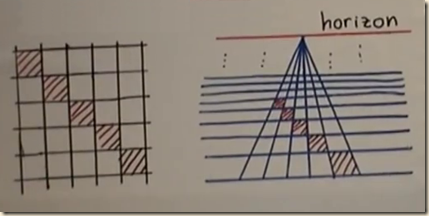(Image source: Wildberger)

The vertical lines meet at the horizon, and the diagonals meet at common points elsewhere on the horizon (above right, not depicted directly). (32.5) Wildberger gives a demonstration where he begins with a quadrilateral shape (pictured most foreground below).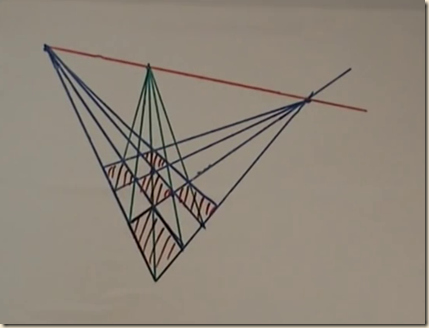(Image source: Wildberger)

The two pairings of opposite sides are extended off to a right and left point on the horizon. The shape’s diagonal lines are set off to a third (in the middle) point on the horizon. Each right/left line creates new points of intersection to draw off to the diagonal horizonal point, which generates new points of intersection for the right/left horizonal lines, and so on as much as needed. (32.6) Rule 3: an image of a conic section is always a conic section. A conic section is formed by intersecting a plane with a cone, with the resulting figure on the plane being the conic section. Depending on the way the plane makes its intersection, you can get a circle, ellipse, hyperbola, parabola, etc.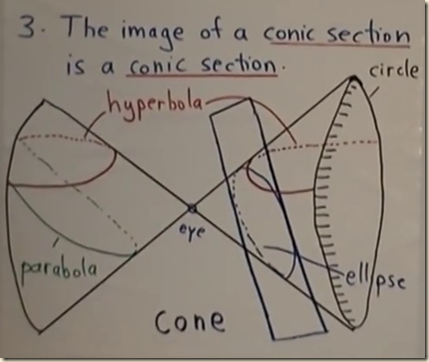(Image source: Wildberger)

We can then apply projective geometry to get images of these sections from a particular point of view, thereby producing a new conic section that has warped on account of the changed perspective on it. (Hence the rule that the image of a conic section is a conic section.) Remarkably then, on account of parallel lines converging at infinity, the image of a parabola conic section from the point of view of the apex can be an ellipse.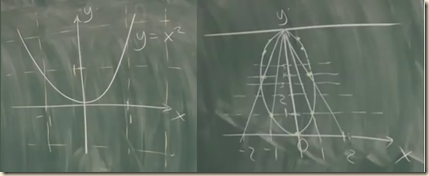(Image source: Wildberger)]

(32.7) If corresponding points on two lines all create additional lines that pass through a common perspective point, then there is a perspectivity between the two lines.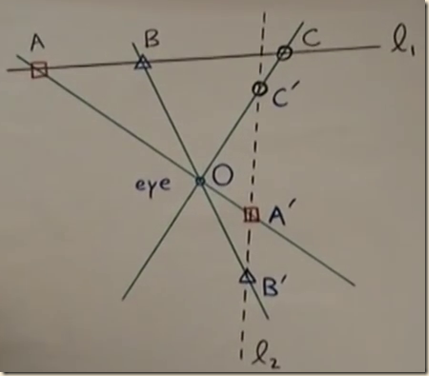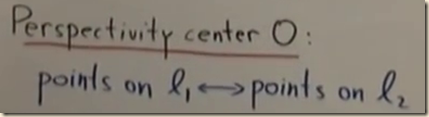(Image source: Wildberger)]

Contents

32.1

Introduction

32.2

An Illustration of Painting Perspective

32.3

Rule 1 of Perspective Painting

32.4

Rule 2 of Perspective Painting

32.5

A Demonstration of Drawing Perspective Lines

32.6

Projected Conic Sections

32.7

Perspectivity of Lines

Summary

32.1

Introduction

(00.05-00.13)

[In this lesson we will examine how projective geometry arose from artists’ work on perspective.]

[ditto]

[contents]

32.2

An Illustration of Painting Perspective

(0o.14-00.53)

[Renaissance painters discovered techniques that implement perspective so to accurately depict the spatial proportions of a three dimensional object upon a two-dimensional surface, as seen from a fixed point of view. The canvas is a two-dimensional “picture plane” set between the artist’s eye and the three-dimensional object placed at a distance. There are lines going from the artist’s eye through the picture plane and to the various parts of the external object. By delineating the depiction according to the places of canvas-intersection of the lines of sight going to the object’s various parts, the object is depicted on the canvas with its proper visual proportions for that perspective.(Image source: Wildberger)]

Wildberger gives some of the historical context. He says that in the 15th century, “artists starting struggling with the idea of how one represents a three-dimensional picture or situation correctly in a two-dimensional picture of it.” He next gives an example. There is a flower in a box (pictured right, below) and an artist’s eye (pictured left, below.)(Image source: Wildberger)

And the artist is painting onto a canvas that is set upon an easel (pictured center, above). And the artist would like to depict the flower in the box, graphically correctly, on the easel. Mathematically, this would involve drawing lines from the eye to the various points on the object (pictured as the dotted lines, above.) And where those lines intersect our picture plane on the canvas, the artist draws the corresponding points (pictured as the image on the easel, above).

[contents]

32.3

Rule 1 of Perspective Painting

(00.54-01.39)

[The Renaissance painters discovered geometrical rules governing perspective painting. Rule 1: The image of a straight line is always a straight line.]

Renaissance artists discovered certain rules that govern this sort of “perspective map” made on the canvas. Rule 1: The image of a straight line is always a straight line.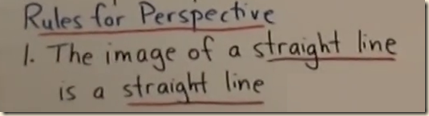(Image source: Wildberger)

Wildberger then illustrates with a diagram.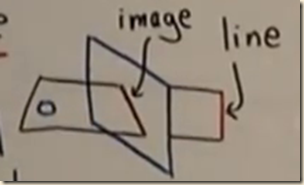(Image source: Wildberger)

We have an eye (pictured as the circle to the left above.) And off in the distance is the line we are trying to represent (pictured right, above). The picture plane is between the two (shown center, above.) We next imagine a plane going from the eye to the line (I think this is the horizontal square above, partly concealed by the picture plane at center). This plane intersects the picture plane. That makes the image of the distant straight line be the image depicted on the picture plane (shown in the vertical square above).

[contents]

32.4

Rule 2 of Perspective Painting

(01.41-03.20)

[Rule 2: images of parallel lines are concurrent (that is, they run together off at infinity upon the horizon). This holds also for diagonal lines, which as well are concurrent off at infinity, and these diagonals set the proportions for spacing out horizontal lines. This is seen for instance when taking a tiled floor (as seen from a bird’s eye view in the below left) and depicting it on a picture plane (seen from the viewer’s perspective, in the below right).(Image source: Wildberger)

The vertical lines meet at the horizon, and the diagonals meet at common points elsewhere on the horizon (above right, not depicted directly).]

The second rule of perspective painting is that images of parallel lines are concurrent.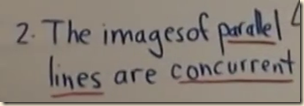(Image source: Wildberger)

This rule arose when dealing with the problem of how to represent a grid of square tiling, like on a floor (pictured left, below) “in a perspective way.”(Image source: Wildberger)

The vertical parallel lines of the floor tiles, as depicted from a bird’s eye view on the left in the above figure, meet at infinity off at the horizon (pictured right, above.) [So it seems that to be concurrent means “runs together”. And what about parallel lines going right and left? Perhaps they run together off on the right and left, but I am not yet sure.] It is like how when we look down train tracks, they appear to narrow as they go off in the distance. But while the fact that vertical lines will meet at the horizon was obvious enough, it was not so obvious how to “stagger” the horizontal lines (that is, how to space them out such that they narrow correctly as they go off in the distance.) The important discovery for this element is that the diagonal lines which are also parallel in the bird’s eye view must from the perspective be concurrent at another point off at infinity (imagine how the diagonal lines of the tiles all extend to the same  horizonal point in the above right diagram).

[contents]

32.5

A Demonstration of Drawing Perspective Lines

(03.22-07.07)

[Wildberger gives a demonstration where he begins with a quadrilateral shape (pictured most foreground below).(Image source: Wildberger)

The two pairings of opposite sides are extended off to a right and left point on the horizon. The shape’s diagonal lines are set off to a third (in the middle) point on the horizon. Each right/left line creates new points of intersection to draw off to the diagonal horizonal point, which generates new points of intersection for the right/left horizonal lines, and so on as much as needed.]

Wildberger will now give a demonstration of how to draw the perspective lines from a given object. We start with the following shape. [It might be a square tile that appears as such from our perspective, but I am not sure.]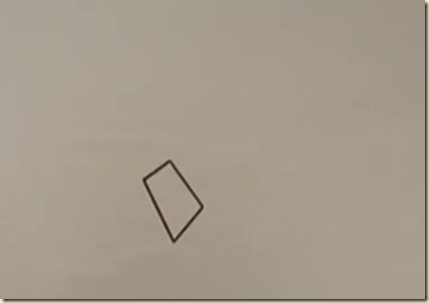(Image source: Wildberger)

Wildberger will extend this quadrilateral to a picture of a square lattice, following the above two rules (see section 32.3 and section 32.4). Straight lines go to straight lines. Here we will extend the left and right side lines for the shape off to a meeting point at infinity on the horizon.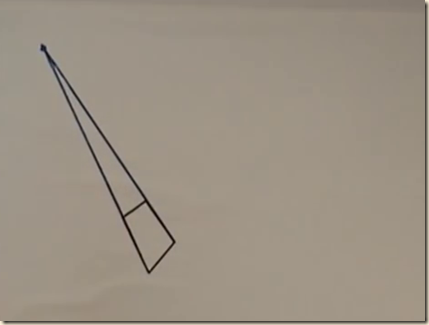(Image source: Wildberger)

Likewise, the other two lines will meet off at infinity at a second point on the horizon.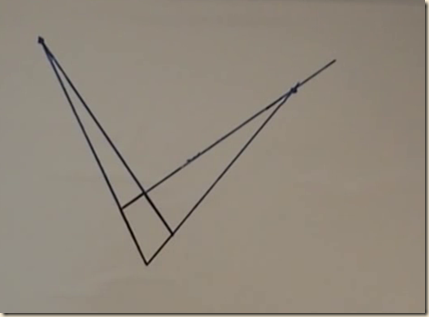(Image source: Wildberger)

With these two points on the horizon, we can now draw the horizon line.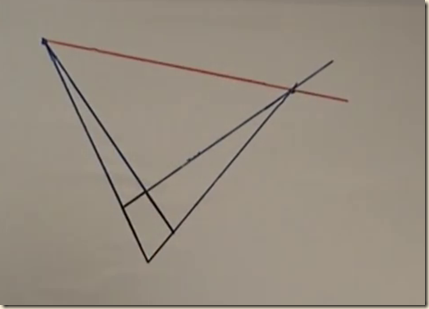(Image source: Wildberger)

We next recall that the shape’s diagonals also meet at the horizon. Here we take the following diagonal and draw it off to the horizon.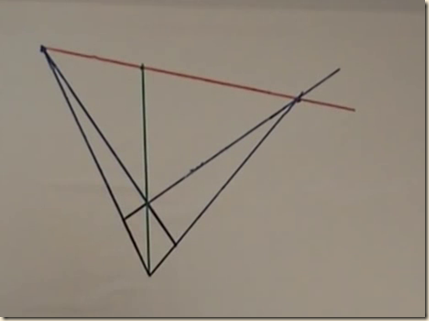(Image source: Wildberger)

This is now a third point on the horizon. All other diagonals will be concurrent to that point on the horizon. We now draw some other lines to that diagonal point (we begin with the lines at intersections of the lines going to the other horizonal points.)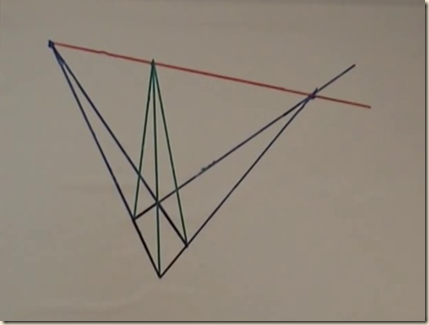(Image source: Wildberger)

This gives us two new points (where the two additional diagonals intersect with lines to the other horizonal points.) These points will be assigned lines going to the right and left horizonal points.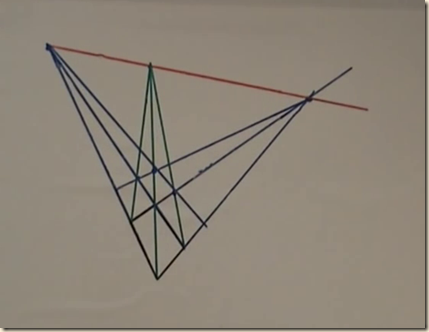(Image source: Wildberger)

This now gives us new points for making new lines to the diagonal horizonal point.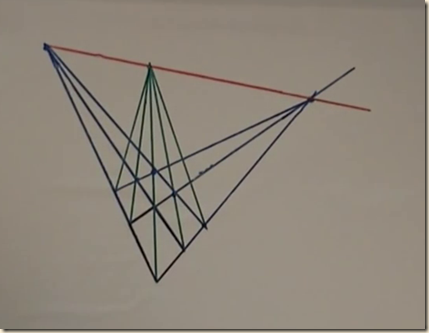(Image source: Wildberger)

This of course gives us new points to connect to the right and left horizonal points.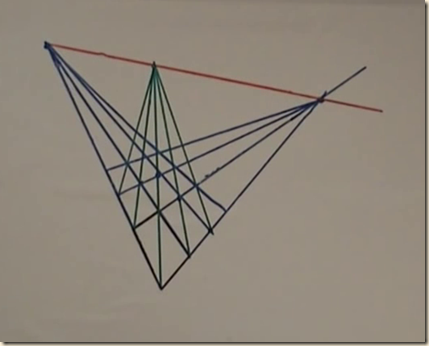(Image source: Wildberger)

This gives us a bunch of different images of our initial square, depicted in a checkerboard pattern.(Image source: Wildberger)

[contents]

32.6

Projected Conic Sections

(07.08-08.24)

[Rule 3: an image of a conic section is always a conic section. A conic section is formed by intersecting a plane with a cone, with the resulting figure on the plane being the conic section. Depending on the way the plane makes its intersection, you can get a circle, ellipse, hyperbola, parabola, etc.(Image source: Wildberger)

We can then apply projective geometry to get images of these sections from a particular point of view, thereby producing a new conic section that has warped on account of the changed perspective on it. (Hence the rule that the image of a conic section is a conic section.) Remarkably then, on account of parallel lines converging at infinity, the image of a parabola conic section from the point of view of the apex can be an ellipse.(Image source: Wildberger)]

The third rule of perspective is that an image of a conic section is always a conic section.(Image source: Wildberger)

For a conic section, we start with an circle (pictured above right) and a vertex (pictured as the central dot above), and we join the circle to the vertex, creating a cone (above right and also left). Then, if we intersect that cone with a plane, that by definition would be a “conic section”. A plane intersecting with the cone as on the right would make an ellipse. A plane intersecting the cone like in the above left and right would make a hyperbola. And if you take a plane that is parallel to one of the sides of the cone, you get a parabola (above left middle). We will now add projective geometry to this schema. We will “take a picture” of a conic section from a particular perspective (in this case, from the “eye” at the center), which will give us normally a different conic section (because it will distort the figure as it is from a birds eye view on it to how it appears from the new point of view. Of course, if the section is a circle, then from the new point of view at the apex/eye, it will also be a circle, because that would be a bird’s eye view on it.) So we suppose we are at the eye, and we are taking a picture of the parabola (left, above). We then make our camera film be the plane on the right, above. We then draw lines from eye to the parabola which give us the points on the film plate (it seems that we extend the lines from the parabola through the eye then to wherever it intersects the plate, giving us a point of the new conic.) This shows us that a picture of a parabola can be an ellipse. Or with a different picture plane, we would get the image of a hyperbola or circle. [A bird’s eye view of the parabola would show a figure that goes on forever outward, with the arms never getting any nearer to one another and certainly meeting. But from a certain point of view on it, the arms will embrace on the other side, closing in on one another. How is that? It is not well explained here, but Wildberger explains it in another video, which we will summarize later. But we can peek at it for now. At around 33.42 minutes into MathHistory8: Projective Geometry.(Image source: Wildberger)

Since the parallel lines are concurrent, that means that even though from a birds eye view the parabola arms go outward, the metrics of its space bend those arms to a point. There is a good drawing of this in Richard Southwell’s video “Projective Geometry 3 When Does A Parabola Look Like An Ellipse?” at around 17.36.]

[contents]

32.7

Perspectivity of Lines

(08.25-end)

[If corresponding points on two lines all create additional lines that pass through a common perspective point, then there is a perspectivity between the two lines.(Image source: Wildberger)]

We will now see that this idea of a perspective applies also to two lines. We begin with our point O, which is our perspective point where our eye is.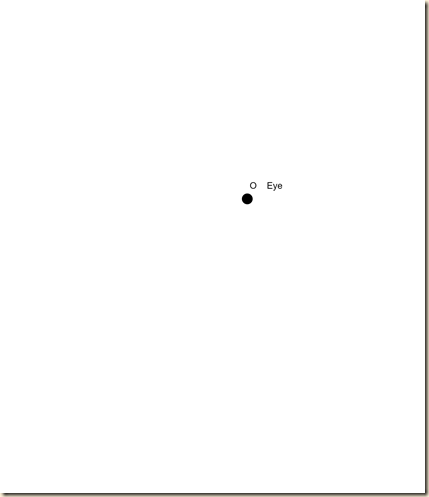And we make a line, ℓ1, with points A, B, C.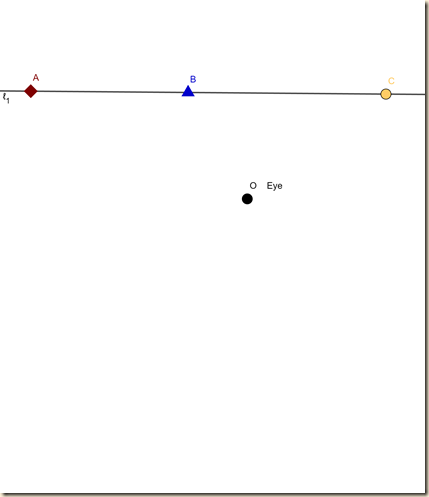We next draw another line ℓ2, with corresponding points B′, A′, C′.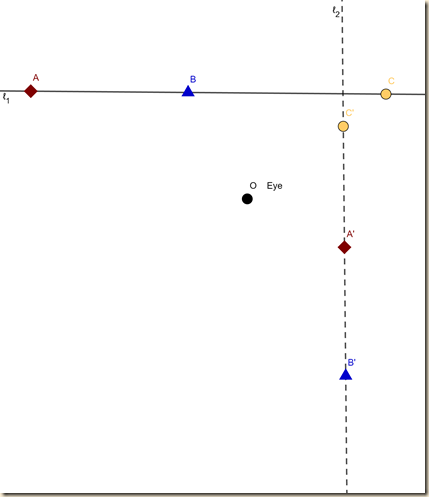Now, these points on ℓ2 are arranged such that perspective lines going through the eye point O connect to the corresponding points on line ℓ1.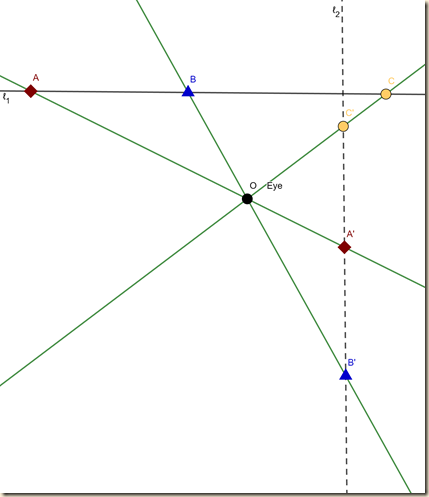(Image source: Wildberger)

When the two lines and their points are such, we say there is a perspectivity between the two lines [with respect to their corresponding points].(Image source: Wildberger)

Wildberger than says that to make that perspectivity one-to-one as a bijection, we need to augment the lines by points at infinity; but he will discuss this more in another lesson. He leaves us with a challenge. We take any line with three points A, B, C. Then we take another line with points A′, B′, C′ spaced out in any way we want [I am not certain if the ordering must be the same, but as we will see, the ordering can vary with the angle of the line]. Regardless of how they are spaced, the second line can be somehow positioned so that the corresponding points fall on the perspective lines going through the perspectivity center. [In Wildberger’s diagram above, the A′ and B′ are set closer to one another than the A′ and C′ are. What if that spacing is inverted, and A′ and B′ are set very far apart and the A′ and C′ are set very near? How could the second line be set in a position for the perspectivity to hold? I would imagine that we would rotate it counter clockwise, which will draw the top two closer and the bottom two further apart, but I am not sure.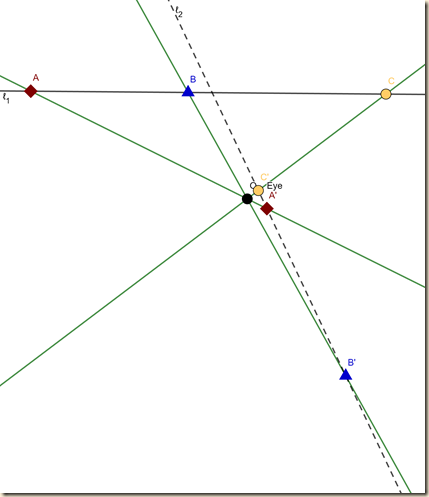And we can change the order of the points too. For instance, we can put B′  in the center.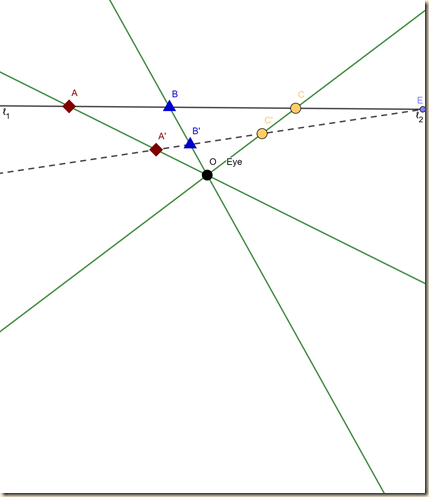Or C′  in the center.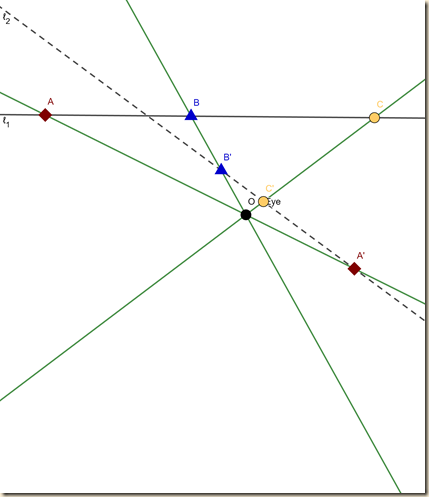]

[contents]

Wildberger, Norman J. (2008) WildTrig: Intro to Rational Trigonometry, 32: “Projective Geometry and Perspective”. Course Series.

Youtube page:

https://youtu.be/qJGmX83MNUY

Course Youtube Playlist:

https://www.youtube.com/watch?v=GGj399xIssQ&list=PL3C58498718451C47

Norman J. Wildberger, youtube channel:

njwildberger

https://www.youtube.com/channel/UCXl0Zbk8_rvjyLwAR-Xh9pQ

Parabola image from:

Wildberger, Norman J. (2011) Math History, 8: “Projective Geometry”. Course Series.

Youtube page:

https://youtu.be/NYK0GBQVngs